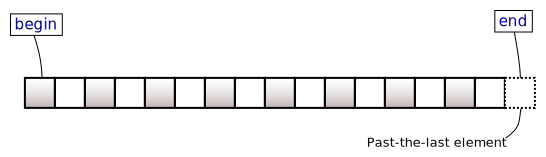/C++

# std::set<Key,Compare,Allocator>::end, std::set<Key,Compare,Allocator>::cend

 (1) `iterator end();` (until C++11) `iterator end() noexcept;` (since C++11) (2) `const_iterator end() const;` (until C++11) `const_iterator end() const noexcept;` (since C++11) `const_iterator cend() const noexcept;` (3) (since C++11)

Returns an iterator to the element following the last element of the `set`.

This element acts as a placeholder; attempting to access it results in undefined behavior.(none).

### Return value

Iterator to the element following the last element.

### Complexity

Constant.

Because both `iterator` and `const_iterator` are constant iterators (and may in fact be the same type), it is not possible to mutate the elements of the container through an iterator returned by any of these member functions.

### Example

```#include <algorithm>
#include <iostream>
#include <set>

int main()
{
std::set<int> set{3, 1, 4, 1, 5, 9, 2, 6, 5};
std::for_each(set.cbegin(), set.cend(), [](int x)
{
std::cout << x << ' ';
});
std::cout << '\n';
}```

Output:

`1 2 3 4 5 6 9`